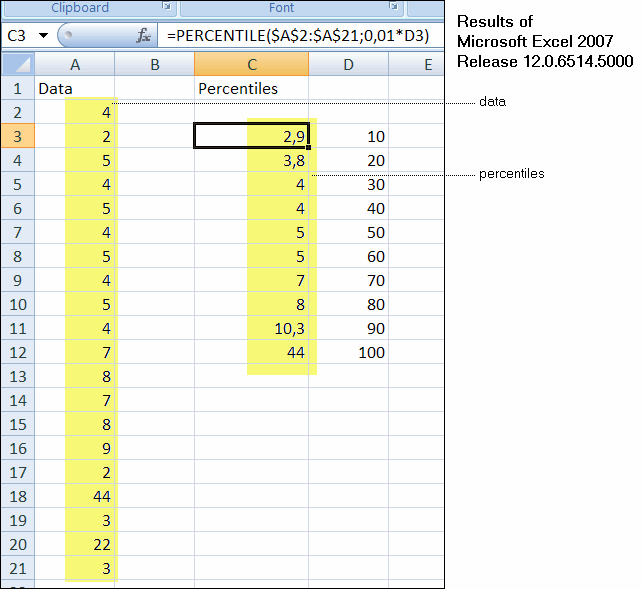## Percentiles Differ from Excel Results

 Problem When calculating the percentiles using the function TDataTable.PercentileOfNumCells the results differ from the results obtained by MicroSoft Excel (the same is true for the functions TVector.Percentile and TMatrix.Percentile). Applies To All versions Solution The mismatch is due to the way how the percentiles are calculated, i.e. if the distribution is interpolated for a given quantile and how it is interpolated. This effect of differing percentile values diminishes when the sample size grows (leading to excactly the same values for infinitely large data sets). Since there are many ways to interpolate (or estimate) a distribution from a small sample, there are as many ways to calculate the quantiles (percentiles). The results of statistical functions of the SDL Component Suite are checked against one of the world's leading statistical program packages, SPSS. As you can see from the following comparison of four statistics packages, the results of the SDL Suite (which is used in DataLab) match the results of SPSS. Both Microsoft Excel and Statgraphics Centurion show different results for the same data set.Last Update: 2010-04-10